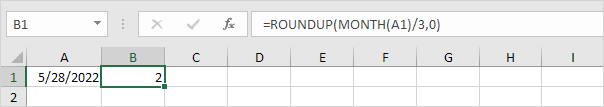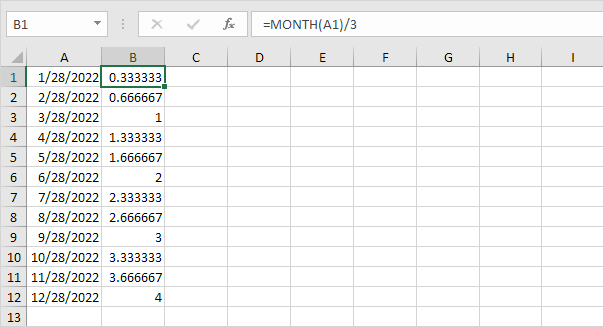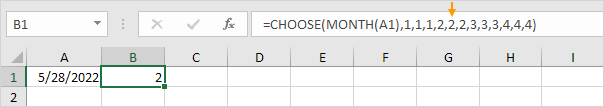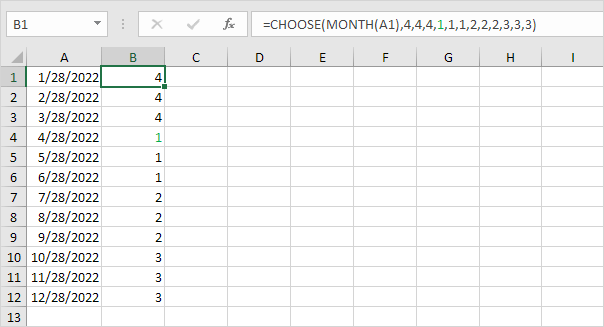# Quarter

An easy formula that returns the quarter for a given date. There's no built-in function in Excel that can do this.

1. Enter the formula shown below.Explanation: ROUNDUP(x,0) always rounds x up to the nearest integer. The MONTH function returns the month number of a date. In this example, the formula reduces to =ROUNDUP(5/3,0), =ROUNDUP(1.666667,0), 2. May is in Quarter 2.

2. Let's see if this formula works for all months.Explanation: now it's not difficult to see that the first three values (months) in column B are rounded up to 1 (Quarter 1), the next three values (months) in column B are rounded up to 2 (Quarter 2), etc.

3. You can also use MONTH and CHOOSE in Excel to return the quarter for a given date.Explanation: in this formula, MONTH(A1) returns 5. As a result, the CHOOSE function returns the fifth choice. May is in Quarter 2.

4. This formula works for all months.Explanation: in this formula, MONTH(A1) returns 1. As a result, the CHOOSE function returns the first choice. January is in Quarter 1.

To return the fiscal quarter for a given date, slightly adjust the list of values.

5. For example, if your company's fiscal year starts in April, use the following formula.Note: green font for illustration only.

6. For example, if your company's fiscal year starts in October, use the following formula.Tip: to quickly copy the formula in cell B1 to the other cells, select cell B1 and double click on the lower right corner of cell B1 (the fill handle).

Go to Next Chapter: Text Functions